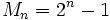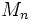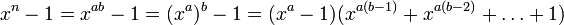Mersenne number is prime implies number is prime

Statement

Supposeis a positive integer such that the Mersenne numberis a prime number. Thenitself is a prime number.

Proof

We prove the statement in its contrapositive form: ifis not prime, thenis not prime. The caseis immediate, so we consider the case, whereby it must be composite.

Given:whereare (possibly equal, possibly distinct) positive integers greater than 1.

To prove:is composite.

Proof: Write. We have a polynomial factorization:Plug inand get:Sinceare both greater than 1,and the other factor are both greater than 1, sois composite.